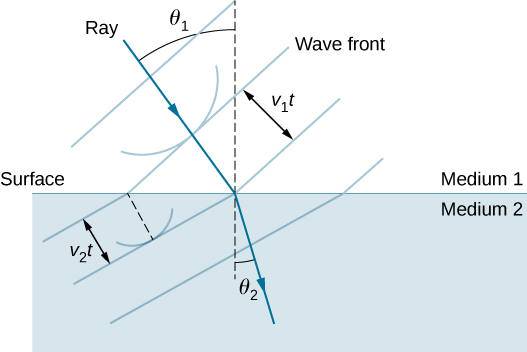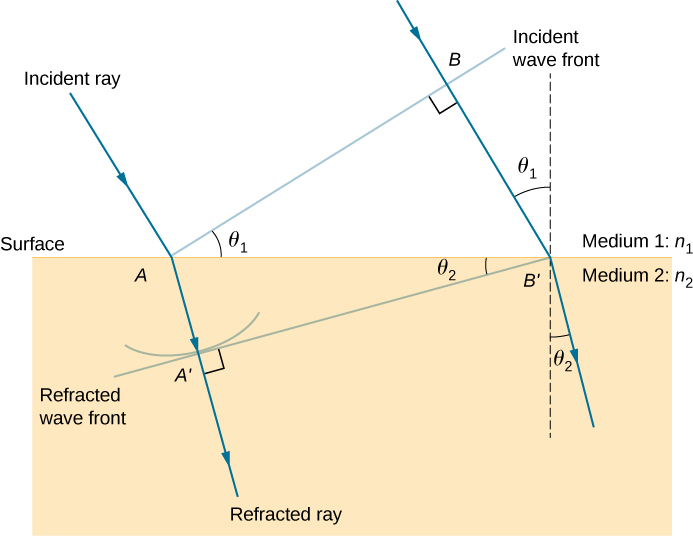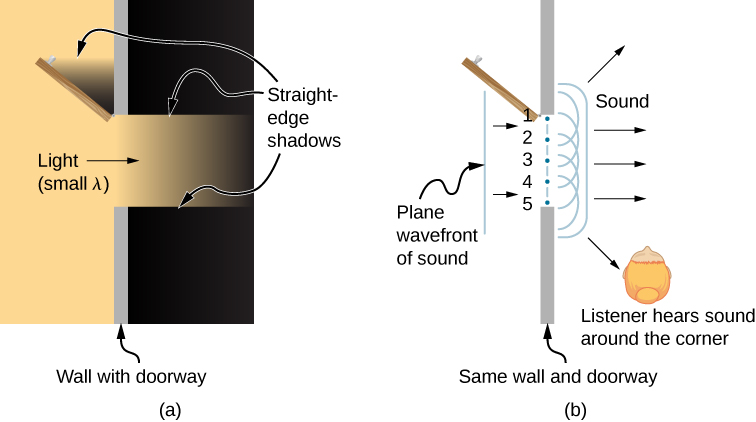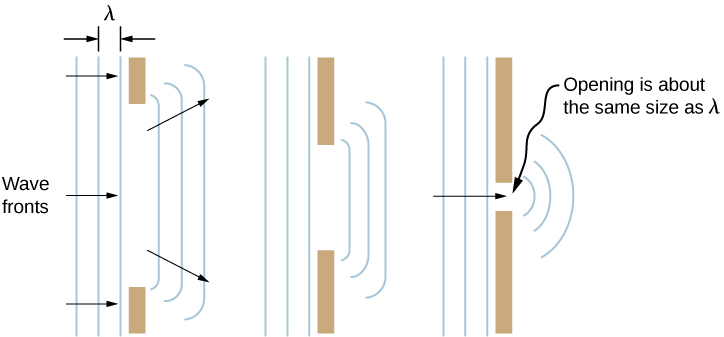# 1.6 Huygens’s principle  (Page 2/4)

 Page 2 / 4

## Refraction

The law of refraction can be explained by applying Huygens’s principle to a wave front passing from one medium to another ( [link] ). Each wavelet in the figure was emitted when the wave front crossed the interface between the media. Since the speed of light is smaller in the second medium, the waves do not travel as far in a given time, and the new wave front changes direction as shown. This explains why a ray changes direction to become closer to the perpendicular when light slows down. Snell’s law can be derived from the geometry in [link] ( [link] ).Huygens’s principle applied to a plane wave front traveling from one medium to another, where its speed is less. The ray bends toward the perpendicular, since the wavelets have a lower speed in the second medium.

## Deriving the law of refraction

By examining the geometry of the wave fronts, derive the law of refraction.

## Strategy

Consider [link] , which expands upon [link] . It shows the incident wave front just reaching the surface at point A , while point B is still well within medium 1. In the time $\text{Δ}t$ it takes for a wavelet from B to reach $B\text{′}$ on the surface at speed ${v}_{1}=c\text{/}{n}_{1},$ a wavelet from A travels into medium 2 a distance of $AA\text{′}={v}_{2}\text{Δ}t,$ where ${v}_{2}=c\text{/}{n}_{2}.$ Note that in this example, ${v}_{2}$ is slower than ${v}_{1}$ because ${n}_{1}<{n}_{2}.$Geometry of the law of refraction from medium 1 to medium 2.

## Solution

The segment on the surface $AB\text{′}$ is shared by both the triangle $ABB\text{′}$ inside medium 1 and the triangle $AA\text{′}B\text{′}$ inside medium 2. Note that from the geometry, the angle $\text{∠}BAB\text{′}$ is equal to the angle of incidence, ${\theta }_{1}$ . Similarly, $\text{∠}AB\text{′}A\text{′}$ is ${\theta }_{2}$ .

The length of $AB\text{′}$ is given in two ways as

$AB\text{′}=\frac{BB\text{′}}{\text{sin}\phantom{\rule{0.2em}{0ex}}{\theta }_{1}}=\frac{AA\text{′}}{\text{sin}\phantom{\rule{0.2em}{0ex}}{\theta }_{2}}.$

Inverting the equation and substituting $AA\text{′}=c\text{Δ}t\text{/}{n}_{2}$ from above and similarly $BB\text{′}=c\text{Δ}t\text{/}{n}_{1}$ , we obtain

$\frac{\text{sin}\phantom{\rule{0.2em}{0ex}}{\theta }_{1}}{c\text{Δ}t\text{/}{n}_{1}}=\frac{\text{sin}\phantom{\rule{0.2em}{0ex}}{\theta }_{2}}{c\text{Δ}t\text{/}{n}_{2}}.$

Cancellation of $c\text{Δ}t$ allows us to simplify this equation into the familiar form

${n}_{1}\phantom{\rule{0.2em}{0ex}}\text{sin}\phantom{\rule{0.2em}{0ex}}{\theta }_{1}={n}_{2}\phantom{\rule{0.2em}{0ex}}\text{sin}\phantom{\rule{0.2em}{0ex}}{\theta }_{2}.$

## Significance

Although the law of refraction was established experimentally by Snell and stated in Refraction , its derivation here requires Huygens’s principle and the understanding that the speed of light is different in different media.

Check Your Understanding In [link] , we had ${n}_{1}<{n}_{2}$ . If ${n}_{2}$ were decreased such that ${n}_{1}>{n}_{2}$ and the speed of light in medium 2 is faster than in medium 1, what would happen to the length of $AA\text{′}$ ? What would happen to the wave front $A\text{′}B\text{′}$ and the direction of the refracted ray?

$AA\text{′}$ becomes longer, $A\text{′}B\text{′}$ tilts further away from the surface, and the refracted ray tilts away from the normal.

This applet by Walter Fendt shows an animation of reflection and refraction using Huygens’s wavelets while you control the parameters. Be sure to click on “Next step” to display the wavelets. You can see the reflected and refracted wave fronts forming.

## Diffraction

What happens when a wave passes through an opening, such as light shining through an open door into a dark room? For light, we observe a sharp shadow of the doorway on the floor of the room, and no visible light bends around corners into other parts of the room. When sound passes through a door, we hear it everywhere in the room and thus observe that sound spreads out when passing through such an opening ( [link] ). What is the difference between the behavior of sound waves and light waves in this case? The answer is that light has very short wavelengths and acts like a ray. Sound has wavelengths on the order of the size of the door and bends around corners (for frequency of 1000 Hz,

$\lambda =\frac{c}{f}=\frac{330\phantom{\rule{0.2em}{0ex}}\text{m/s}}{1000\phantom{\rule{0.2em}{0ex}}{\text{s}}^{-1}}=0.33\phantom{\rule{0.2em}{0ex}}\text{m,}$

about three times smaller than the width of the doorway).(a) Light passing through a doorway makes a sharp outline on the floor. Since light’s wavelength is very small compared with the size of the door, it acts like a ray. (b) Sound waves bend into all parts of the room, a wave effect, because their wavelength is similar to the size of the door.

If we pass light through smaller openings such as slits, we can use Huygens’s principle to see that light bends as sound does ( [link] ). The bending of a wave around the edges of an opening or an obstacle is called diffraction. Diffraction is a wave characteristic and occurs for all types of waves. If diffraction is observed for some phenomenon, it is evidence that the phenomenon is a wave. Thus, the horizontal diffraction of the laser beam after it passes through the slits in [link] is evidence that light is a wave. You will learn about diffraction in much more detail in the chapter on Diffraction .Huygens’s principle applied to a plane wave front striking an opening. The edges of the wave front bend after passing through the opening, a process called diffraction. The amount of bending is more extreme for a small opening, consistent with the fact that wave characteristics are most noticeable for interactions with objects about the same size as the wavelength.

## Summary

• According to Huygens’s principle, every point on a wave front is a source of wavelets that spread out in the forward direction at the same speed as the wave itself. The new wave front is tangent to all of the wavelets.
• A mirror reflects an incoming wave at an angle equal to the incident angle, verifying the law of reflection.
• The law of refraction can be explained by applying Huygens’s principle to a wave front passing from one medium to another.
• The bending of a wave around the edges of an opening or an obstacle is called diffraction.

## Conceptual questions

How do wave effects depend on the size of the object with which the wave interacts? For example, why does sound bend around the corner of a building while light does not?

Does Huygens’s principle apply to all types of waves?

yes

If diffraction is observed for some phenomenon, it is evidence that the phenomenon is a wave. Does the reverse hold true? That is, if diffraction is not observed, does that mean the phenomenon is not a wave?

what is bohrs model for hydrogen atom
hi
Tr
Hello
Youte
Hi
Nwangwu-ike
hi
Siddiquee
what is the value of speed of light
1.79×10_¹⁹ km per hour
Swagatika
what r dwarf planet
what is energy
কাজের একক কী
Jasim
কাজের একক কী
Jasim
friction ka direction Kaise pata karte hai
friction is always in the opposite of the direction of moving object
Punia
A twin paradox in the special theory of relativity arises due to.....? a) asymmetric of time only b) symmetric of time only c) only time
b) symmetric of time only
Swagatika
fundamental note of a vibrating string
every matter made up of particles and particles are also subdivided which are themselves subdivided and so on ,and the basic and smallest smallest smallest division is energy which vibrates to become particles and thats why particles have wave nature
Alvin
what are matter waves? Give some examples
according to de Broglie any matter particles by attaining the higher velocity as compared to light'ill show the wave nature and equation of wave will applicable on it but in practical life people see it is impossible however it is practicaly true and possible while looking at the earth matter at far
Manikant
a centeral part of theory of quantum mechanics example:just like a beam of light or a water wave
Swagatika
Mathematical expression of principle of relativity
given that the velocity v of wave depends on the tension f in the spring, it's length 'I' and it's mass 'm'. derive using dimension the equation of the wave
What is the importance of de-broglie's wavelength?
he related wave to matter
Zahid
at subatomic level wave and matter are associated. this refering to mass energy equivalence
Zahid
it is key of quantum
Manikant
how those weight effect a stable motion at equilibrium
how do I differentiate this equation- A sinwt with respect to t
just use the chain rule : let u =wt , the dy/dt = dy/du × du/dt : wA × cos(wt)
Jerry
I see my message got garbled , anyway use the chain rule with u= wt , etc...
Jerry
de broglie wave equation
vy beautiful equation
chandrasekhar
what is electro statics
when you consider systems consisting of fixed charges
SherlyBy Anonymous UserByBy Hoy WenBy Jonathan LongBy Jill ZerressenBy Edgar DelgadoBy OpenStaxBy OpenStaxBy Rohini AjayBy OpenStax